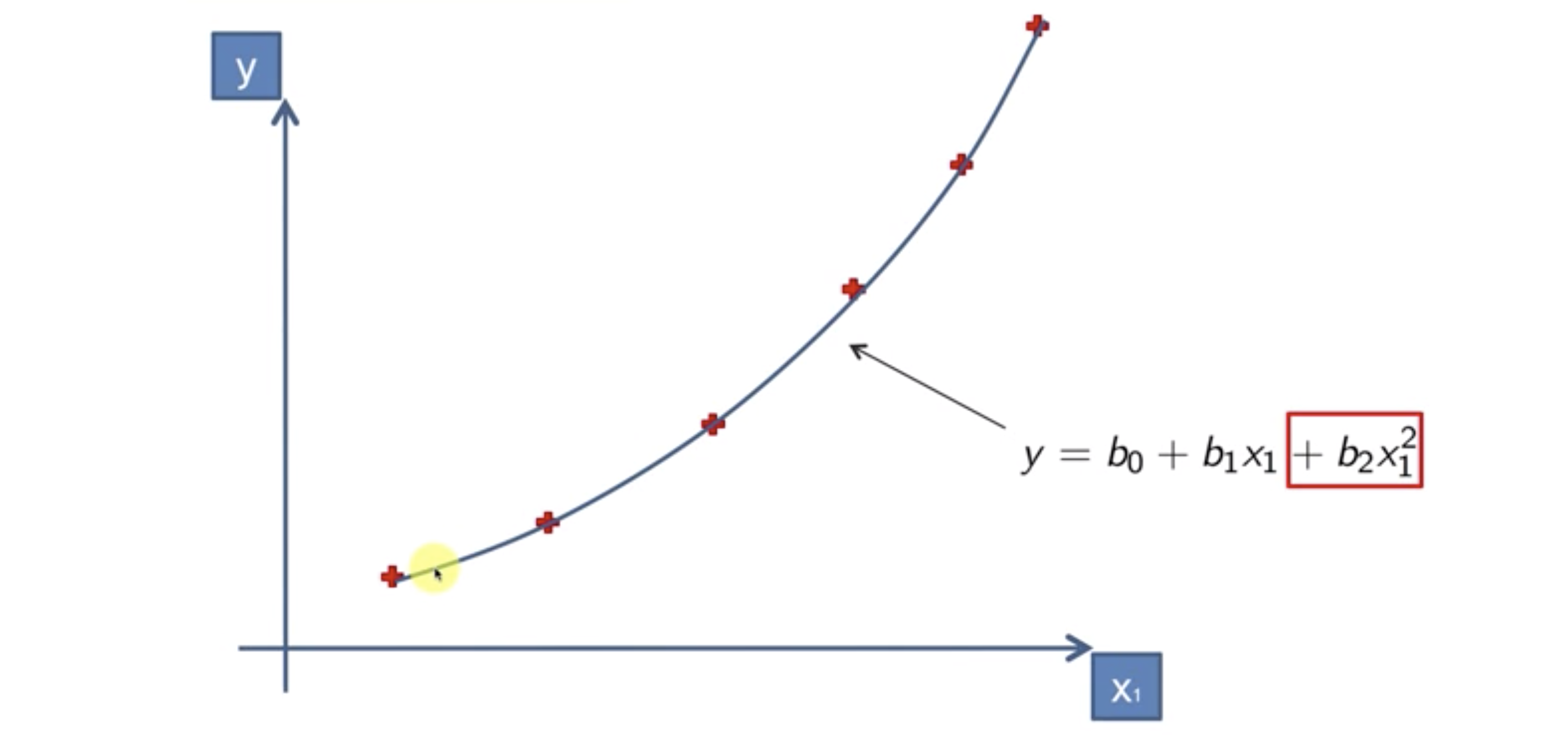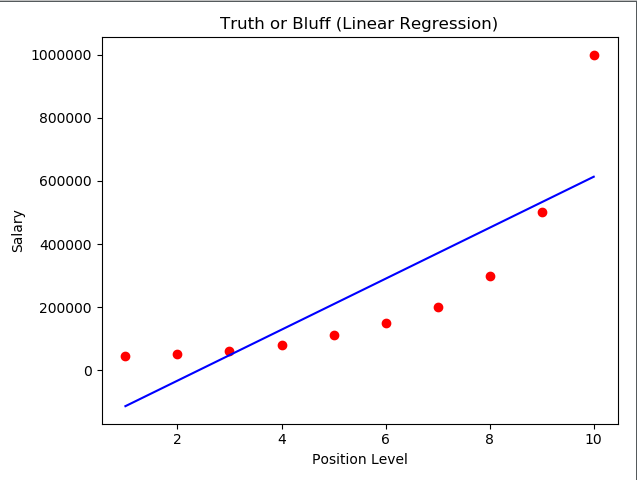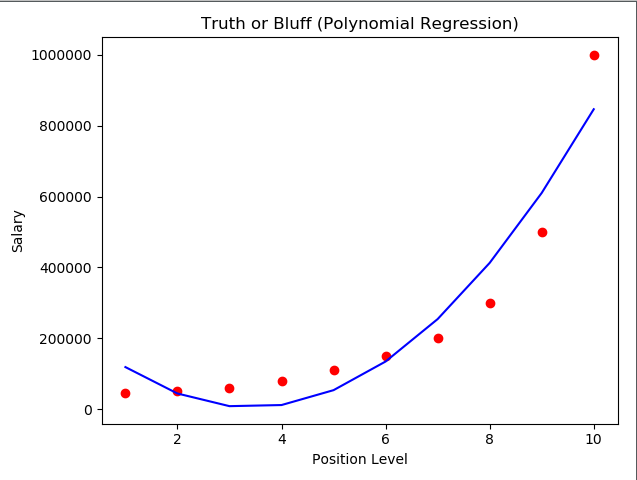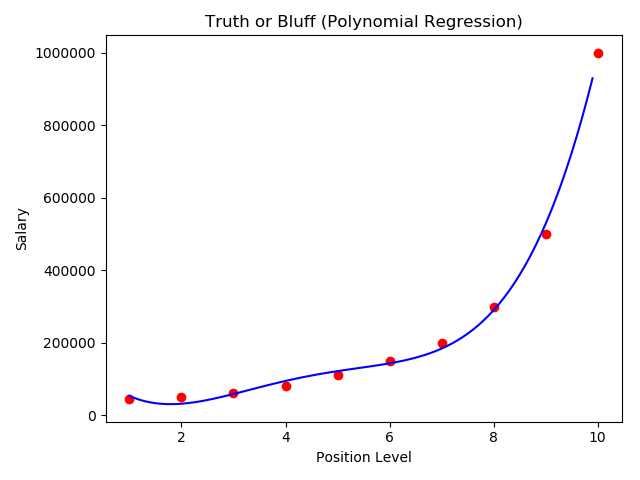y = b_0 + b_1x_1 + b_2x_1^2 + … + b_nx_1^nPosition Level Salary
Junior Consultant 2 50000
Senior Consultant 3 60000
Manager 4 80000
Country Manager 5 110000
Region Manager 6 150000
Partner 7 200000
Senior Partner 8 300000
C-level 9 500000
CEO 10 1000000

import pandas as pd
from sklearn.linear_model import LinearRegression
from sklearn.preprocessing import PolynomialFeatures

data_path = '../data/Position_Salaries.csv'

X = dataset.iloc[:, 1:2].values
y = dataset.iloc[:, 2].values


# Fitting Linear Regression to the Training set
lin_reg = LinearRegression()
lin_reg.fit(X, y)


# Fitting Ploynomial Regression to the Training set
poly_reg = PolynomialFeatures(degree=2)
X_poly = poly_reg.fit_transform(X)
lin_reg2 = LinearRegression()
lin_reg2.fit(X_poly, y)


# Visualising the Linear Regression results
plt.scatter(X, y, c='r')
plt.plot(X, lin_reg.predict(X), c='b')
plt.title('Truth or Bluff (Linear Regression)')
plt.xlabel('Position Level')
plt.ylabel('Salary')
plt.show()
# Visualising the Polynomial Regression results
plt.scatter(X, y, c='r')
plt.plot(X, lin_reg.predict(X), c='b')
plt.plot(X, lin_reg2.predict(poly_reg.fit_transform(X)), c='b')
plt.title('Truth or Bluff (Polynomial Regression)')
plt.xlabel('Position Level')
plt.ylabel('Salary')
plt.show()X_grid = np.arange(min(X), max(X), 0.1)
X_grid = X_grid.reshape(len(X_grid), 1)
plt.scatter(X, y, c='r')
plt.plot(X_grid, lin_reg2.predict(poly_reg.fit_transform(X_grid)), c='b')
plt.title('Truth or Bluff (Polynomial Regression)')
plt.xlabel('Position Level')
plt.ylabel('Salary')
plt.show()


# Predicting a new result with Linear Regression
lin_reg.predict(6.5)

# Predicting a new result with Polynomial Regression
lin_reg2.predict(poly_reg.fit_transform(6.5))